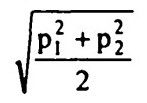top of pageSearch

# Strength of Materials(21-40)

Updated: Apr 8, 202021.The weakest section in a diamond riveting as shown in Fig. 10.1 is

section 1 - 1

22. According to Unwin's formula, the diameter of rivet in mm to suit the t mm

thickness of plate is given by23.A flat carrying a pull of 690 kN is con nected to a gusset plate using rivets. If the

pulls required to shear the rivet, to crush the rivet and to tear the plate per pitch

length are 68.5 kN, 46 kN and 69 kN respectively, then the number of rivets

required is 15

24.If the rivet value is 16.8 kN and force in the member is 16.3 kN, then the number

of rivets required for the connection of the member to a gusset plate is 225.In the riveted connection shown in Fig.10.2, which of the rivets will be subjected

to maximum stress ?

rivets B and D

26.When a member is subjected to axial ten sile load, the greatest normal stress is

equal to twice the maximum shear stress

27.At a point in a strained body carrying two unequal unlike principal stresses pi and p2 (Pi > P2), the maximum shear stress is given by (p, + p2)/2

28. If a point in a strained material is subjected.to equal normal and tangential

stresses, then the angle of obliquity is 45°

29. If the principal stresses at a point in a strained body are pi and p2 (pi > P2), then the resultant stress on a plane carrying the maximum shear stress is equal to30. The plane of maximum obliquity is inclined to the major principal plane at an

anglewhere Φ max is the angle of maximum obliquity.

31.A point in a strained body is subjected to a tensile stress of 100 MPa on one plane and a tensile stress of 50 MPa on a plane at right angle to it. If these planes are carrying shear stresses of 50 MPa, then the principal stresses are inclined to the larger normal stress at an angle32.If a prismatic member with area of cross section A is subjected to a tensile load P, then the maximum shear stress and its inclination with the direction of load

respectively are P/2A and 45°

33.The sum of normal stresses is constant

34.The radius of Mohr's circle for two equal unlike principal stresses of magnitude p is p

35.Shear stress on principal planes is zero

36.The state of pure shear stress is produced by tension in one direction and equal

compression in perpendicular direction

37.A prismatic bar is carrying only an axial force. The two planes on which normal

and shearing stresses are equal are inclined to the axial force at angles38.According to Rankine's hypothesis, the criterion of failure of a brittle material is

maximum principal stress

39.Maximum bending moment in a beam occurs where shear force changes sign

40.A simply supported beam of span l carries over its full span a load varying linearly from zero at either end to w/unit length at midspan. The maximum bending moment occurs at

midspan and is equal to